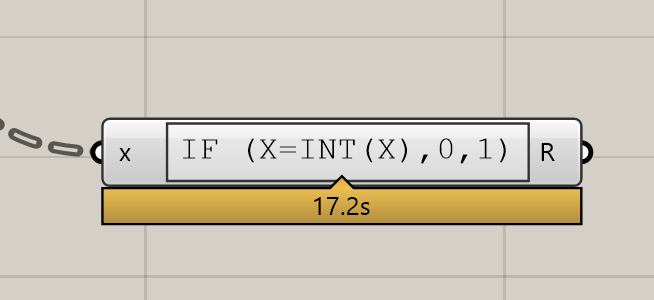# How to check if a number is integer

I tried this way, but I’m looking for a faster way to the computer. Here I have entered a million entries and it takes a long time.
Thank you.And this waytestint.gh (7.4 KB)

`````` private void RunScript(List<object> dbls, ref object ints)
{
const double Tolerance = RhinoMath.ZeroTolerance;

var output = new List<int>();
var cnt = dbls.Count;
for(var i = 0; i < cnt;i++)
{
if(dbls[i] is double)
{
var num = (double) dbls[i];
var rounded = (int) Math.Round(num, MidpointRounding.AwayFromZero);

if(Math.Abs(num - rounded) < Tolerance)
{
}
}
}
ints = output;
}``````
2 Likes

I was goingto say, 7.3s isn’t actually that bad. Of course Keyu Gan’s C# answer is pretty performant, but if you are dealing with 1 million values and doing purely mathematical operations, GH is probably not the right tool.

What type of thing are you creating that has a million values? I’m really curious.

But using the parameter components to convert data types is always rather slow, so try to avoid that.

1 Like

This is for the Euler project. Thank you

1 Like

its perfect!!!

It might also be useful to note that e.g. the Python `float` class has the `is_integer()` method. And that a general method across languages is to use the modulus operator:

210318_ModulusIntegerTest_Python.gh (6.1 KB)

Edit: Although it will never be faster than C#, here’s a more performant implementation:

210318_ModulusIntegerTest_Python_01.gh (6.2 KB)

2 Likes

Project Euler is a great way to learn to code, whether it is with Excel, GrassHopper, Python, C# …

I expect you will quickly find that GrassHopper is too slow as you reach the larger, harder problems - I used python to solve a lot of problems, generally lookiing for algorithms which solve in a few seconds or less.

-Graham2 Likes

It would be more inefficient if there’re tons of integers in the list because Python doesn’t handle the conversion from `float` to `int` and leave it to GH, which is slow.

That’s not accurate, assuming I understood you correctly. You can explicitly cast the output to Grasshopper integers in GHPython:

Sad to see how much faster this computes in Rhino 5 by the way1 Like

The best I can do:

``````namespace PancakeAlgo.Misc
{
public class pcFilterInt : GH_Component
{
private static FieldInfo _field;

static pcFilterInt()
{
_field = typeof(GH_Structure<GH_Integer>).GetField("m_data", BindingFlags.Instance | BindingFlags.NonPublic);
}

/// <summary>
/// Initializes a new instance of the pcFilterInt class.
/// </summary>
public pcFilterInt()
: base("pcFilterInt", "pcFilterInt",
"Description",
"Pancake", "Algorithm")
{
}

/// <summary>
/// Registers all the input parameters for this component.
/// </summary>
protected override void RegisterInputParams(GH_Component.GH_InputParamManager pManager)
{
}

/// <summary>
/// Registers all the output parameters for this component.
/// </summary>
protected override void RegisterOutputParams(GH_Component.GH_OutputParamManager pManager)
{
}

/// <summary>
/// This is the method that actually does the work.
/// </summary>
/// <param name="DA">The DA object is used to retrieve from inputs and store in outputs.</param>
protected override void SolveInstance(IGH_DataAccess DA)
{
const double tolerance = RhinoMath.ZeroTolerance;
DA.GetDataTree<IGH_Goo>(0, out var numbers);
var tree = new GH_Structure<GH_Integer>();

var path = new GH_Path(0);

tree.EnsurePath(path);
var list = new List<GH_Integer>();

foreach (IGH_Goo obj in numbers)
{
if (obj is GH_Number it)
{
if (it is null) continue;
var val = it.Value % 1;
if (val > -tolerance && val < tolerance)
{
}
}
else if (obj is GH_Integer @int)
{
}
}

var internalData = (SortedList<GH_Path, List<GH_Integer>>)_field.GetValue(tree);
internalData[path] = list;
DA.SetDataTree(0, tree);
}
}
}
``````

You can. I mean casting it in Python is faster. (not quite in my demo coz there’s only one integer)

2 Likes